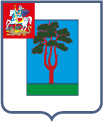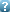Landau Days 2015 Landau Days 2015 June, 22-25 Chernogolovka, RussiaJosephson current between topological and conventional superconductors Date/Time: 14:00 22-Jun-2015 Abstract: We study the stationary Josephson current in junctions between a topological superconductor and a conventional, topologically trivial superconductor. Such an S-S$_{top}$ junction hosts a Majorana zero mode. The Josephson effect in such an S-S$_{top}$ junction is more complicated than in conventional junctions due to broken time-reversal symmetry. We derive general formulae for the current and bound state spectrum for a class of short topological junctions. The result is strongly asymmetric with respect to the superconducting gaps $\Delta_{triv}$ and $\Delta_{top}$ in the trivial and topological superconductor. In addition to the Majorana zero mode characteristic of an S-S$_{top}$ junction, the junction may or may not host additional sub-gap bound states, depending on parameters. We apply our general formulae to a simple nanowire-based setup. In the tunnelling limit the critical current behaves as $I_c\propto \Delta_{top}/R_N^2$. This is in strong contrast with the Ambegaokar-Baratoff relation: current scales as conductance squared, and only depends on the topological gap $\Delta_{top}$. Authors Ioselevich Pavel A(Presenter) (no additional information) © 2012, Landau Institute for Theoretical Physics RAS www.itp.ac.ru Contact webmaster